## Wednesday, May 16, 2012

### The Nexus of Corruption and Income Part V

[Warning: this post is highly graphics intensive]

Last month, I did a series on the relationship between corruption measures and income levels and growth which you might want to revisit before reading through this post (Links: Part I, Part II, Part III, and Part IV). Reader Shihong asked if there’s any differences to the relationships if we subdivide the dataset by income levels. The short answer is, yes there is, and the differences are pretty interesting too.

To recap, the corruption measure used here is from Transparency International’s Corruption Perception Index (CPI) from 1995 to 2011, which captures through surveys the perception of corruption in each country on a scale ranging from zero (totally corrupt) to 10 (corruption free). GDP data is sourced from the IMF September 2011 World Economic Outlook database, in PPP adjusted current international dollars.

What follows are the charts tabulating GDP per capita and GDP per capita growth against the CPI score across the whole dataset: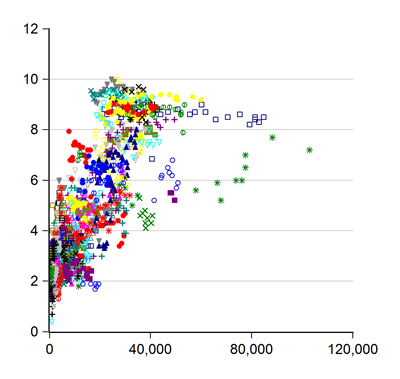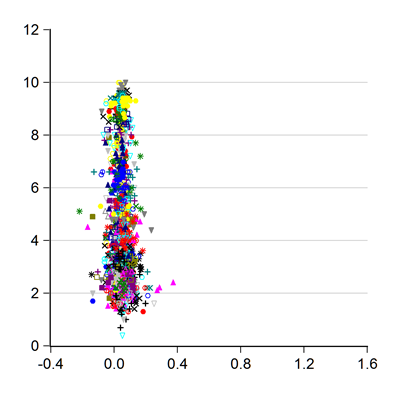Both charts have the CPI score on the vertical axis; the top chart has GDP per capita on the horizontal axis, while the bottom chart uses real GDP per capita growth. The first chart demonstrates that there is a correlation between the CPI and the GDP per capita level, but the second chart shows that there is no systematic relationship between economic growth and corruption levels – which would be necessary if reducing corruption is a precondition of reaching higher income levels…or vice-versa.

Is there however, a difference between different income levels? Let’s start at the bottom and move up to the top.

I’m using here the latest country income classification from the World Bank to subdivide the dataset. Technically this isn’t quite correct, as some countries have “graduated” to different income levels across time, but adjusting for that is more work than I’m willing to spend on this. If anyone wants to make the attempt, the original dataset is here, while the country groupings are here.

As above, the following charts have CPI against GDP per capita on top, and against GDP growth at the bottom. I’m also including the unbalanced panel regression results for each section (GDP level against CPI, CPI against GDP level, GDP growth against CPI, CPI against GDP growth; standard errors in brackets; p-values are also reported for the dependent variable coefficient), with a summing up at the bottom of the post. For reference, Malaysia is classified as an upper middle income country.

Low Income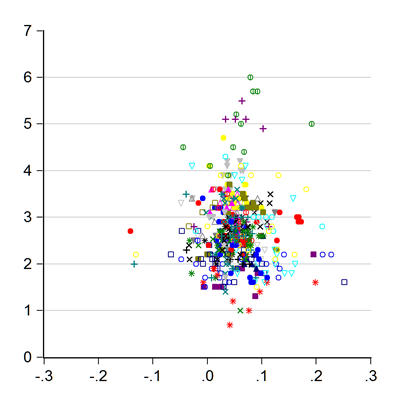log GDP = 6.70 (0.06) + 0.20 (0.07) * log CPI (p=0.003)

log CPI = –0.31 (0.39) + 0.17 (0.06) * log GDP (p=0.003)

GDP growth = 0.03 (0.19) + 0.01 (0.01) * CPI (p=0.399)

CPI = 2.39 (0.03) + 0.43 (0.51) * GDP growth (p=0.399)

Lower Middle Income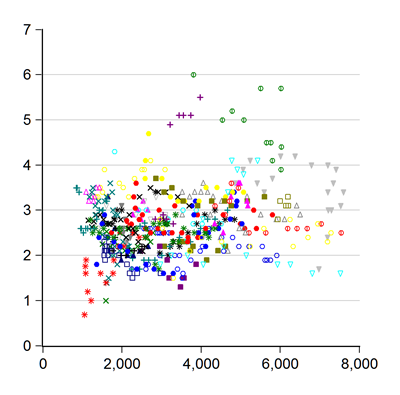log GDP = 7.72 (0.07) + 0.26 (0.07) * log CPI (p=0.0003)

log CPI = 0.08 (0.24) + 0.11 (0.03) * log GDP (p=0.0003)

GDP growth = 0.07 (0.01) - 0.00 (0.01) * CPI (p=0.381)

CPI = 2.74 (0.03) - 0.36 (0.42) * GDP growth (p=0.381)

Upper Middle Income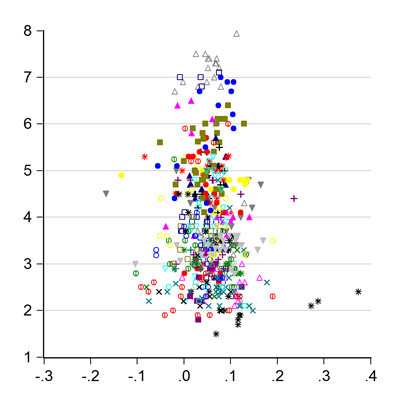log GDP = 8.82 (0.13) + 0.17 (0.10) * log CPI (p=0.091)

log CPI = 0.98 (0.18) + 0.03 (0.02) * log GDP (p=0.091)

GDP growth = 0.06 (0.02) - 0.00 (0.00) * CPI (p=0.801)

CPI = 3.83 (0.03) - 0.10 (0.41) * GDP growth (p=0.801)

High Income: Non-OECD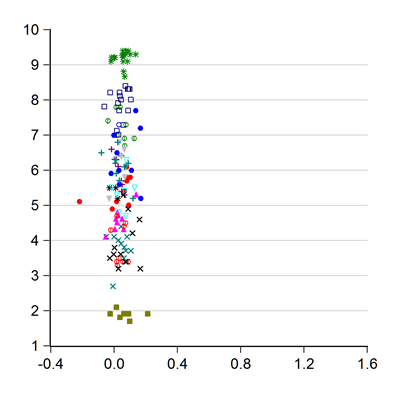log GDP = 10.16 (0.28) + 0.04 (0.17) * log CPI (p=0.7985)

log CPI = 1.58 (0.47) + 0.01 (0.05) * log GDP (p=0.7985)

GDP growth = -0.01 (0.06) + 0.01 (0.01) * CPI (p=0.289)

CPI = 5.81 (0.05) + 0.83 (0.78) * GDP growth (p=0.289)

High Income: OECD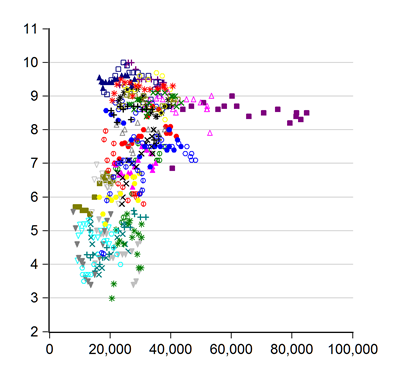log GDP = 9.34 (0.23) + 0.43 (0.12) * log CPI (p=0.0003)

log CPI = 1.30 (0.18) + 0.06 (0.02) * log GDP (p=0.0003)

GDP growth = 0.00 (0.02) + 0.01 (0.00) * CPI (p=0.086)

CPI = 7.16 (0.03) + 1.16 (0.67) * GDP growth (p=0.086)

Commentary

Overall, the breakdowns by income level show the same general results as the overall dataset – there exists a correlation between corruption scores and income level, which disappears when using GDP growth instead.

But there’s a couple of exceptions – for upper middle income countries, the correlation between GDP level and the CPI is weak (p-value=0.0909), and for non-OECD high income countries, the correlation doesn’t exist at all (p-value=0.7985).

[Digression: what’s a p-value? It’s the probability that the estimated coefficient is generated by chance and not a reflection of the “true” population value;  the lower the number, the more confident you are that the result is statistically significant. (1 – p-value) actually gives the significance level, e.g. for a p-value=0.0909, the significance level is 90.91%]

Actually, eyeballing the scatter plots, the only income segment where I’m fully confident that the correlation actually exists in reality is in the OECD high income segment. Looking at the other income segments, it’s hard to be really sure that the panel regressions are capturing a true correlation, or being overly influenced by data outliers.

Another interesting factoid that stands out is that in the high income segments (both OECD and non-OECD), the CPI range is really, really wide – actually that’s largely true for the upper middle income bracket as well.

High income OECD CPI scores range from 3 to nearly 10, while for the non-OECD, it ranges from about 2 to a little over 9. The upper middle income countries have CPI scores ranging from 2 to 8. It’s only in the low income and lower middle income countries that we find low CPI scores and low income levels coinciding. Translation: high income levels are not systematically related to low corruption.

So what’s the bottom line? I stand by my earlier conclusion that there’s no systematic relationship between corruption and income levels. The breakdowns presented here really reinforce that, especially given the wide range of CPI scores even for high income countries.

I would put two qualifiers however – countries relying on natural resource extraction can skew the results, because you can attain high income levels without being efficient or showing good governance (e.g. the Middle East). Secondly, taking into account drifts over time in income classification for individual countries might also result in different conclusions. Quite a few countries change income classifications each year.

Taking these into account however would take a lot of work, and I’m not sure if the results would turn out any different.

Technical Notes:

1. Corruption data is from Transparency International’s Corruption Perception Index, from 1995 to 2011
2. GDP per capita data is taken from the IMF World Economic Outlook database (September 2011) – series code PPPPC.
3. Country Income Classification from the World Bank

1.wat up bro

why don't u link ur other post on the Shadow Economy to this...

2.Hey bro,

It never occurred to me. But that's an interesting proposition (the link between shadow economies, corruption and income).

3.Looks like pretty damning evidence to me - the non-correlation, I mean.

But I wonder what the scatter plot would look like if you take the CPI vs. potential output - the hypothesis being that the actual losses to corruption would have an impact on a country's potential. I think only OECD countries have those, and I think BNM has done recent work for ours, in their annual report (can't reveal my in-house office one, sorry).

My guess is that if you can strip out cyclical effects (unlikely), there might be some relationship. Again, that's a guess.

4.Thanks hishamh for the update. Much appreciated.

5.@Jason,

I doubt using potential output would make that much of a difference. Under "normal" circumstances, the output gap wouldn't be very big. In any case, some types of corruption are supposedly growth positive, which makes it even harder to tease out any relationship.

@Shihong,

You're welcome.

6.That's conjecture though.

I'm actually believe that corruption does not influence growth, but there's not enough evidence (to me) to disprove that statement to everyone else.

Oh a little bit of nitpicking, I think I read somewhere on the TI website that the CPI does not use a consistent methodology year-to-year, since its mostly surveys and questionnaires anyway. So I think a time series representation may not be enough to quiet down the cynics.